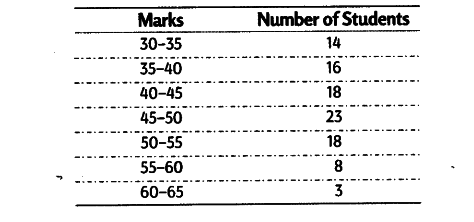# Calculate the value of median, first quartile and third quartile from the following data

Calculate the value of median, first quartile and third quartile from the following data.